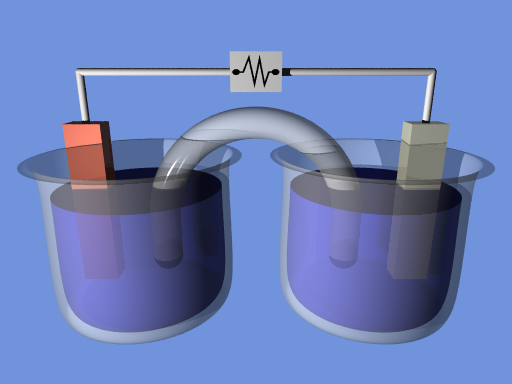## The Nernst Equation

#### Learning Objective

• Recall the Nernst equation

#### Key Points

• In electrochemistry, the Nernst equation can be used to determine the reduction potential of a half-cell in an electrochemical cell.
• The Nernst equation can also be used to determine the total voltage (electromotive force) for a full electrochemical cell.
• The Nernst equation gives a formula that relates the numerical values of the concentration gradient to the electric gradient that balances it.

#### Terms

• electromotive forceVoltage generated by a battery or by a varying magnetic field.
• voltageThe amount of electrostatic potential between two points in space.
• electrochemical cellA container containing an electrolyte and two electrodes; used to produce direct current electricity. One or more of them constitute a battery.
• electrochemistryThe science of the chemistry associated with the flow of electricity, especially at the surface of an electrode.

In electrochemistry, the Nernst equation can be used, in conjunction with other information, to determine the reduction potential of a half-cell in an electrochemical cell. It can also be used to determine the total voltage, or electromotive force, for a full electrochemical cell. It is named after the German physical chemist who first formulated it, Walther Nernst.Electrochemical cellSchematic of an electrochemical cell.

The Nernst equation gives a formula that relates the electromotive force of a nonstandard cell to the concentrations of species in solution:

$E = E^o - \frac {0.0257}{n} ln\ Q$

In this equation:

• E is the electromotive force of the non-standard cell
• Eo is the electromotive force of the standard cell
• n is the number of moles of electrons transferred in the reaction

ln Q is the natural log of $\frac{C^cD^d}{A^aB^b}$, where the uppercase letters are concentrations, and the lowercase letters are stoichiometric coefficients for the reaction: $aA + bB \rightarrow cC + dD$

## Example

Find the cell potential of a galvanic cell based on the following reduction half-reactions where [Ni2+] = 0.030 M and [Pb2+] = 0.300 M.

Ni2+ + 2 e- → Ni, E0 = -0.25 V

Pb2+ + 2 e- → Pb, E0 = -0.13 V

First, find the electromotive force for the standard cell, which assumes concentrations of 1 M.

In order for this reaction to run spontaneously (positive Eo cell) the nickel must be oxidized and therefore its reaction needs to be reversed. The added half-reactions with the adjusted E0 cell are:

$Pb^{2+} + Ni \rightarrow Ni^{2+} + Pb, \ \ \ E^o = 0.12\ V$

The number of moles of electrons transferred is 2 and Q is $\frac{[Ni^{2+}][Pb]}{[Pb^{2+}][Ni]}$, where Pb and Ni are pure solids whose concentrations remain constant, so they are dropped from the equation.

$E = E^o - \frac {0.0257}{n} ln\ Q$

$E = 0.12V - \frac {0.0257}{2} ln\frac{0.030}{0.300}$

$E = 0.15\ V$